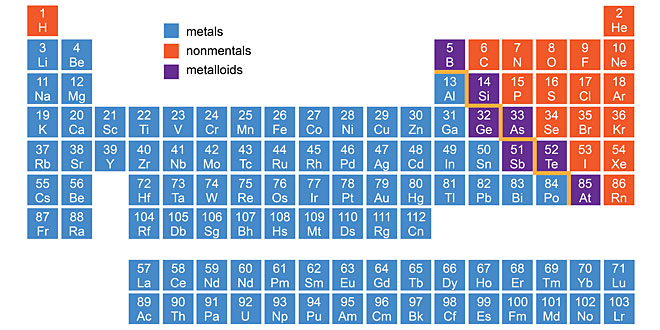Tuesday , August 20 2019
Home / 10th Class / Metals and Non-metals: 10th Science# Metals and Non-metals: 10th Science

Answer:

1. Sodium
2. Mercury
3. Gallium
4. Iodine
5. Carbon

#### Question: What happens when: (1). A piece of calcium is dropped in water. (2). Sodium is kept exposed in air. (3). Iron nail is dipped in copper sulphate solution. (4). Potassium is dropped in cold water. (5). Carbon dioxide is compressed in water at high pressure.

Give chemical equation involved in all the above reactions and balance them.

Answer:

1. Calcium starts floating because the bubbles of hydrogen gas formed stick to its surface.
Ca(s) + 2H2O(l) → Ca(OH)2(aq) + H2(g)
2. In dry air, sodium forms sodium peroxide.
4Na(s) + O2(g) → 2Na2O2(s)
(Sodium peroxide)
If the air is moist and contains a lot of water, sodium reacts violently with the water content of air forms sodium hydroxide and hydrogen gas, producing a lot of heat
2Na(s) + 2H2O(l) → 2NaOH(s) + H2(g) + Heat
3. Iron displaces copper from copper sulphate solution.
Fe + CuSO4  → FeSO4  +  Cu
4. Potassium reacts violently with cold water.
2K(s) + 2H2O(l) → 2KOH(aq) + H2(g) + Heat energy
5. Carbonic acid is formed.
CO2  + H2O → H2CO3.

#### Question: Write chemical equation when zinc granules react with: (1). Sulphuric acid (2). Hydrochloric acid (3). Aluminium chloride (4). Sodium hydroxide (5). Nitric acid

Answer:

1. Zn + H2SO4  → ZnSO4 + H2
2. Zn + 2HCl → ZnCl2 + H2
3. Zn + AlCl2  → No reaction
4. Zn + 2NaOH → Na2ZnO2 + H2
5. Zn + 4HNO3  → Zn(NO3)2 + 2NO2 + 2H2O

#### Question: Give reasons Why: (1). Ionic compounds have high melting and boiling points. (2). Ionic compounds are soluble in water. (3). Ionic compounds are solid and somewhat hard. (4). Ionic compounds conduct electricity in molten state. (5). The metals have tendency to lose electrons.

Answer:

1. Ionic compounds have high melting and boiling points because a considerable amount of energy is required to break the strong inter-ionic attraction.
2. Ionic compounds are soluble in water because their oppositely charged ions break by the opposite pole water molecules.
3. They are solids and are somewhat hard because of the strong force of attraction between the positive and negative ions.
4. Ionic compound conduct electricity in molten state because the electricity in molten state because the electrostatic forces of attraction between the oppositely charged ions are overcome due to heat.
5. Metals lose electrons as a tendency to attain a completely filled valence  shell.

#### Question: Name the metal which (1) become blackish after certain time. (2) develops green coating on exposure to air. (3) does not corrode at all. (4) is used in making steel. (5) is non-lustrous.

Answer:

1. Silver
2. Copper
3. Platinum
4. Nickel, Chromium
5. Sodium

#### Question: A metal ‘E’ is stored under kerosene oil. When a small piece of it is left open in the air, it catches fire. When the product formed is dissolved in water it turns red litmus blue. (i) Name the metal E. (ii) Write the chemical equation for the reaction when it is exposed to air and when the product is dissolved in water. (iii) Explain the process by which the metal is obtained from its molten chloride.

Answer: (i) Sodium
(ii) 4Na + O→ 2Na2O
Na2O + H2O → 2NaOH
(iii)
Sodium is obtained from sodium chloride by the process of electrolytic reduction.
Reactions: At cathode: Na+ + e→ Na
At anode: 2Cl → Cl2  + 2e

#### Question: Give reason for the following: (1). Gold and platinum are used in jewellery. (2). Copper cannot displace hydrogen from dilute acids. (3). Stainless steel does not easily. (4). Metals an be given different shapes according to our needs. (5). Zinc does not give hydrogen gas on reacting with HNO3.

Answer:

1. They are shiny and unreactive.
2. Copper is lesser reactive than hydrogen.
3. As it is an alloy.
4. Malleable and ductile.
5. As HNOis a strong oxidising agent and oxidises H2 to H2O.

## Gender Religion Caste: 10th Pol Science

Question: What do you mean by sexual division of labour? Answer: A system in which all …